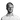10 JavaScript Oneliners You Have Got to Add Your Arsenal as a Developer

JavaScript

29 November, 2020 • ☕️ 2 min read

JavaScript can do a lot of amazing things!

From complex frameworks to handling API’s, there is just SO much to learn.

But, it also enables you to do some awesome stuff using just one single line.

Check out these 10 oneliners to step up your JavaScript game!

1. Capitalize the first letter of a stringUsing this function, you’ll be able to capitalize the first letter of the input of a string. This could be a single word, but also an entire sentence.

// Capitalize the first letter of a string
const capitalize = str => `\${str.charAt(0).toUpperCase()}\${str.slice(1)}`;

capitalize("hello, you are a cool person!");
// Result: "Hello, you are a cool person!"

2. Calculate the number of days between two datesThis can be a little tricky to figure out sometimes. The “1000 * 60 * 60 * 24” is the number of milliseconds that are in a single day.

// Calculate the number of days between two dates
const diffDays = (date, otherDate) => Math.ceil(Math.abs(date - otherDate) / (1000 * 60 * 60 * 24));

diffDays(new Date('2014-12-19'), new Date('2020-01-01'));
// Result: 1839

3. Convert a string to a numberA very straight forward way of converting a string to a number using type coercion.

// Convert a string to a number implicitly
toNumber = str => +str;

// Convert a string to a number explicitly
toNumber = str => Number(str);

toNumber("2");
// Result: 2

4. Check if an array contains any itemsUsing the “isArray” method and checking if the length of the array is higher than 0, we can check if it is empty.

// Check if an array contains any items
const isNotEmpty = arr => Array.isArray(arr) && arr.length > 0;

isNotEmpty([1, 2, 3]);
// Result: true

isNotEmpty([]);
// Result: false

5. Different ways of merging multiple arraysThere are a couple of different ways of merging arrays. One of them is using the “concat” method. Another one is using the spread operator (“…”).

We can also rid the final array of any duplicates using the “Set” object.

// Different ways of merging multiple arrays

// Merge but don't remove the duplications
const merge = (a, b) => a.concat(b);
// Or
const merge = (a, b) => [...a, ...b];

// Merge and remove the duplications
const merge = [...new Set(a.concat(b))];
// Or
const merge = [...new Set([...a, ...b])];

6. Sort an array containing numbersJavaScript is very tricky when it comes to its built-in sort method. It doesn’t handle numbers well, so this function is a simple way of sorting your array.

// Sort an array containing numbers
const sort = arr => arr.sort((a, b) => a - b);

sort([1, 5, 2, 4, 3]);
// Result: [1, 2, 3, 4, 5]

7. Generate a random HEX colorGenerating an RGB color is a little more simple, but creating a random HEX color can get a little complex. This function will allow you to generate a random HEX color.

// Generate a random HEX color
randomColor = () => `#\${Math.random().toString(16).slice(2, 8).padStart(6, '0')}`;

// Or
const randomColor = () => `#\${(~~(Math.random()*(1<<24))).toString(16)}`;Know the name of a cookie and want to read out its specific value?

We can use the “cookie” property of the document object to retrieve it and return it back to us.

// Get the value of a specified cookie

// Result: "GA1.2.1929736587.1601974046"

9. Swap the values of 2 variablesSometimes, you just need to swap 2 variables around. This simple trick will allow you to do that in a single line!

// Swap the values of 2 variables
let a = 1;
let b = 2;

[a, b] = [b, a];
// Result:
// a = 2
// b = 1

10. Get the text that the user has selectedWhenever a user has selected text using the cursor, we can access it using “getSelection” method on the window object.

// Get the text that the user has selected
const getSelectedText = () => window.getSelection().toString();

getSelectedText();

Thanks for reading! I hope you learned something new today.

Want to improve your JS skills even more? Check out my other article “6 Essential JavaScript Tips for the Developer of 2020” to get even better at JS.

Have a nice day! 😄